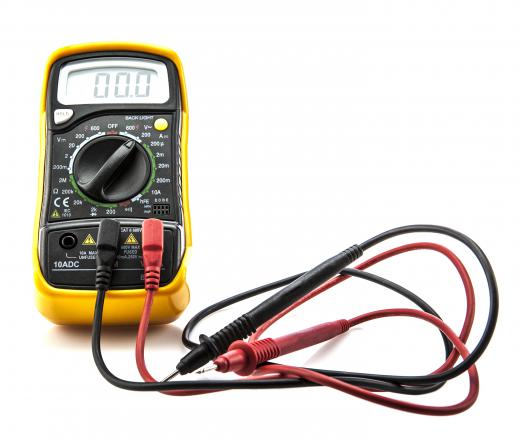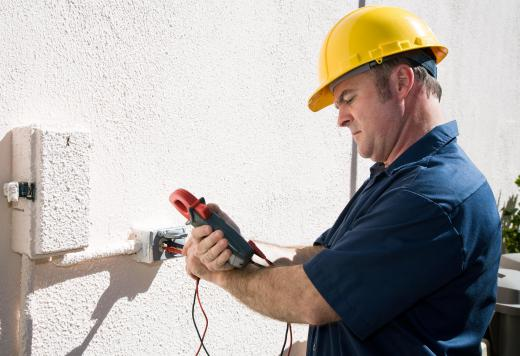Industry
Fact Checked

# What is a Current Meter?

J.M. Densing
J.M. Densing

A current meter, often referred to as an ammeter, is a device that measures the flow of electrical current in a circuit. Current meters can be digital or analog and are frequently part of a larger multimeter that can measure voltage and resistance as well. These devices — both current and multimeters — are often rectangular and box-like in shape, with either a digital display or analog gauge, several dials or switches, and one red and one black wire extending from it.

When referring to electricity, current is the action of electrical charge or electrons flowing through wires that make up a circuit. The rate of the current's movement can be measured using a meter. Current flows in one direction, usually from positive to negative, and it needs to flow in a circuit, which is like a continuous loop. It flows more easily through some materials, such as metal wire, than others. Wood, for example, does not conduct electricity at all.Also known as an ammeter, a current meter measures the flow of electricity in a circuit.

Electric current is measured in a unit called amperes, abbreviated as "amps" or simply "A," by using a current meter. When measuring current, the meter is actually measuring how many electrons pass a specific point each second. In order for the current to be measured it must flow through the meter, which is accomplished by making the current meter part of the circuit.Current meters can help electricians figure out if there is a problem with an electric device.

The meter has two wires coming from it, with probes or clamps attached to the ends that connect to the circuit. To do this, the circuit needs to be broken in the place where the current will be measured, then the red wire is either clamped or touched to the side of the wire leading back to the positive end of the power source, and the black is connected to the negative side. Once the meter is placed within the circuit in this way, it will give a reading.

The reading obtained by a current meter is useful in several ways. By measuring current, it is often possible to determine if a circuit is operating correctly. The measurement can tell if enough electricity is flowing, or if there may be a problem with the power source. If current is measured near a device such as a light or radio, it can be possible to tell if the proper amount of current is flowing into or through the device. This can help a person figure out if there is a problem with the device itself, or if the circuit or power source is to blame.

## You might also Like